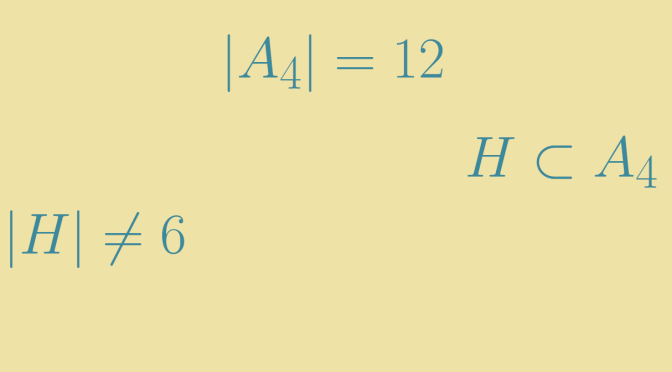Converse of Lagrange’s theorem does not hold

Lagrange’s theorem, states that for any finite group $$G$$, the order (number of elements) of every subgroup $$H$$ of $$G$$ divides the order of $$G$$ (denoted by $$\vert G \vert$$).

Lagrange’s theorem raises the converse question as to whether every divisor $$d$$ of the order of a group is the order of some subgroup. According to Cauchy’s theorem this is true when $$d$$ is a prime.

However, this does not hold in general: given a finite group $$G$$ and a divisor $$d$$ of $$\vert G \vert$$, there does not necessarily exist a subgroup of $$G$$ with order $$d$$. The alternating group $$G = A_4$$, which has $$12$$ elements has no subgroup of order $$6$$. We prove it below.

$$G$$ consists of:

• The identity or neutral element $$e$$.
• The three elements that are product of disjoint transpositions. Those $$3$$ elements with $$e$$ make up a subgroup $$V \subset H$$ ($$V$$ is isomorphic to the Klein four-group)
• The eight 3-cycles.

Suppose that $$H \subset G$$ is a subgroup of order $$6$$ and that $$H^\prime$$ denotes the intersection $$H \cap V$$. $$H^\prime$$ is a subgroup of $$H$$ and $$V$$. By Lagrange’s theorem, $$H^\prime$$ order divides $$4$$ and $$6$$. So $$\vert H^\prime \vert$$ is equal to $$1$$ or to $$2$$. If $$\vert H^\prime \vert=1$$, the map $$(h,v) \mapsto h \cdot v$$ defined from $$H \times V$$ to $$G$$ is one-to-one. Which doesn’t make sense as $$G$$ would have at least $$24$$ elements. Therefore $$\vert H^\prime \vert=2$$ and $$H$$ is made up the identity $$e$$, an element $$v$$ which is product of two disjoint transpositions and six 3-cycles.

Also the index $$\vert G : H\vert$$ is equal to $$2$$ and consequently $$H$$ is a normal subgroup of $$G$$. We recall the argument. For $$a \in G \setminus H$$ the left cosets $$H, aH$$ form a partition of $$G$$. Similarly, the right cosets $$H, Ha$$ form a partition of $$G$$. As $$aH \neq H$$, we have $$aH =Ha$$ which allows to conclude.

We denote $$v=(i,j)(k,l)$$ with $$\{i,j,k,l\} = \{1,2,3,4\}$$ and $$t$$ the 3-cycle $$(i,j,k)$$. We have $$tvt^{-1}=(j,k)(i,l) \neq (i,j)(k,l)$$ and $$tvt^{-1} \in H$$ as $$H \triangleleft G$$. In contradiction with the cardinality of $$\vert H^\prime \vert = \vert H \cap V \vert= 2$$. We have finally proven that $$A_4$$ has no subgroup of order $$6$$.